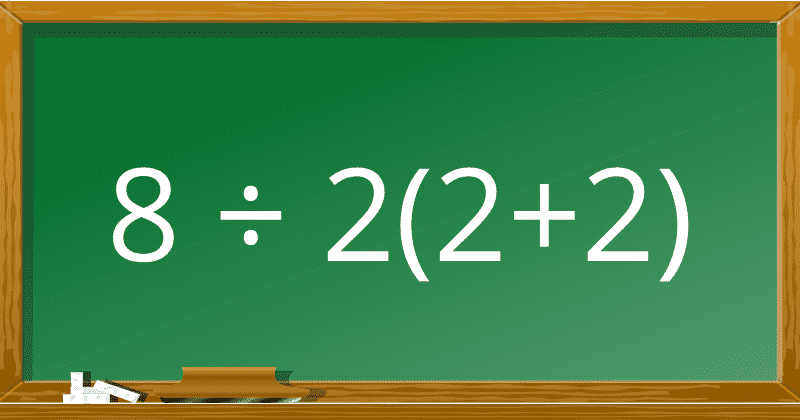Fun Test: Can You Solve This Math Question That Left the Internet Divided?

# Fun Test: Can You Solve This Math Question That Left the Internet Divided?

It sure is confusing.On July 28, 2019, a Twitter user posed a question that looked rather simple, but the internet couldn't seem to come to a conclusion on the answer(s). The question was to solve "8 ÷ 2(2+2)".

While many people were sure the answer was 16, others argued that it was 1. Soon enough, people began the trash talk, and even then, there was a lot of confusion on what the final answer would be.

According to New York Times, everyone on Twitter agreed that the 2+2 within the brackets, aka the parenthesis, should be evaluated first. That's what you were taught in school, too, right?  So, 2+2 is 4, which means the question boils down to 8÷2×4.

But you see, here's where it gets complicated. Now that we’re faced with a division and a multiplication, which one takes priority? If we carry out the division first, we get 4×4 = 16; if we carry out the multiplication first, we get 8÷8 = 1.

Given that there are two answers to one question, which one is actually right? Apparently, the standard convention is that both multiplication and division have equal priority.

Citing PEMDAS (Parenthesis, Exponents, Multiplication, Division, Addition, Subtraction)or BODMAS (Bracket, Of, Division, Multiplication, Addition, and Subtraction), some claimed that the order of operations did not necessarily demand multiplication to happen first and then division. They explained how multiplication and division have an equal hierarchy and that one can solve them in whichever order they appear in an equation.

To break the tie, we work from left to right. So the division goes first, followed by multiplication. If this is the rule applied, then 16 is the right answer. Generally, the conventional order of operations is to evaluate expressions in parentheses first.

Then, the exponents are dealt with, followed by multiplication and division, which are considered to have equal priority, with ambiguities dispelled by working from left to right. Finally come addition and subtraction, which are also of equal priority, with ambiguities broken again by working from left to right, per New York Times.

Since there's still no clarity on the way to answer the confusing question, an all-out brawl broke out between the ones and the 16s, which even got Berkeley to admit that it was a poorly written equation and that even they were uncertain about the correct answer.

References:

https://www.nytimes.com/2019/08/02/science/math-equation-pedmas-bemdas-bedmas.html

https://math.berkeley.edu/~gbergman/misc/numbers/ord_ops.html

Cover Image Source: Canva